Walid Asmar, July 21, 2017 | 2 min read

### Ruby’s Spaceship Operator <=>Although quite simple, Ruby’s spaceship operator can seem confusing and overwhelming for anyone who has never used it. So in this blog post, I hope to not only show you how to use it, but also how it works when sorting elements in an array. The code for this post was executed in the irb, so you can and should follow along with your irb.

If you aren’t familiar with Ruby’s spaceship operator, <=>, it compares two objects and returns 1 if the first object’s value is larger, 0 if both values are equal, and -1 if the second object’s value is larger.

If we’re comparing 2 numbers it looks like this:

`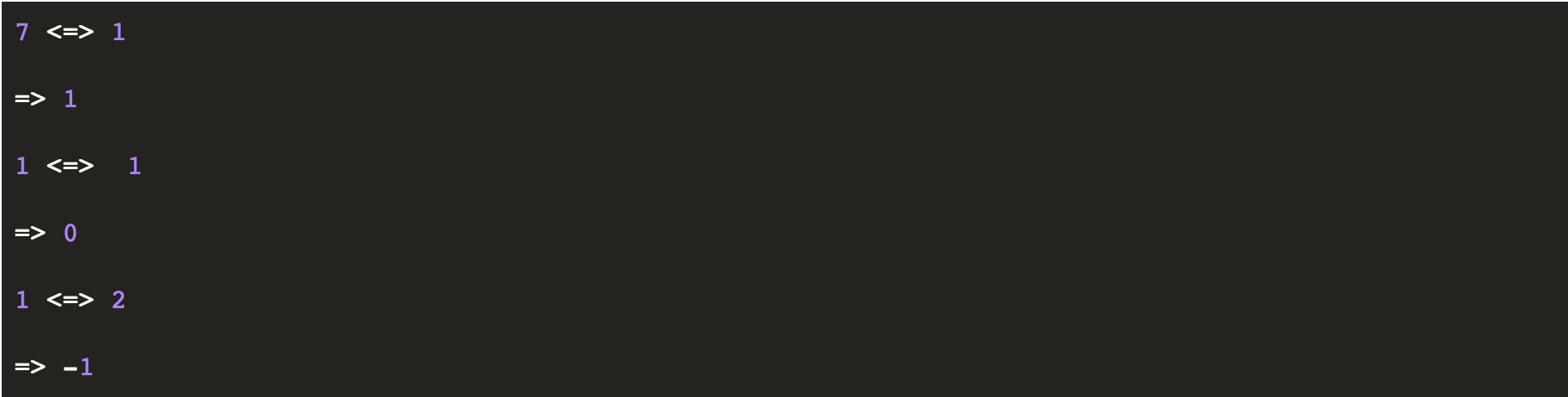`

In our first example it compared 7 to 1 and returned 1 because 7 has a larger value. In our second example 0 was returned because the values are equal, and in our final example it returned -1 because 2 is larger than 1. Pretty self-explanatory!

### Comparing Strings

The spaceship operator can also compare strings. This is where a lot of people get tripped up. However, the important thing to remember is that the operator compares strings in ASCII order. So:

`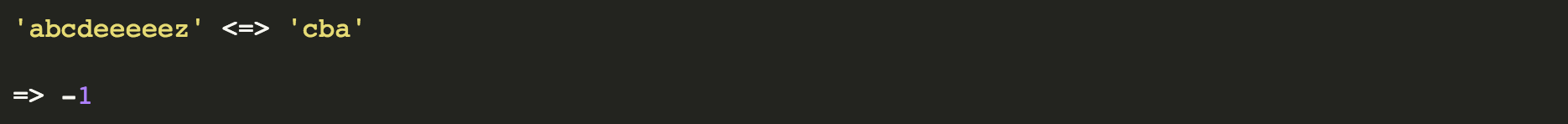`

In the example above, notice the operator returned -1 even though the first string is longer than the second. This is because c has a higher value than a and the spaceship operator will not evaluate past that.

If you need to evaluate strings by length, you just have to add .length after each string. That’ll look like:

`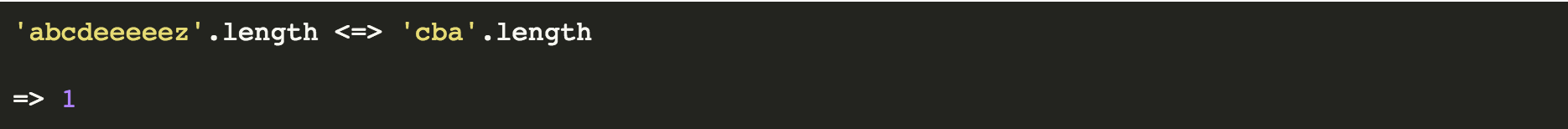`

Another important note for strings is to remember lowercase characters have higher values than their uppercase counterparts.

`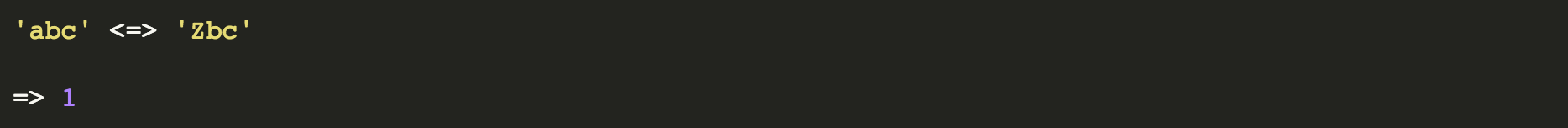`

In the above example the operator returned 1 because lowercase a has a higher value than capital Z, even though lowercase z has a higher value than lowercase a.

### Sorting Elements in an Array with <=>

Probably the most useful way to use the spaceship operator is inside of a block.

Below we have an array named arr. We’re using the sort method to sort the array based on values. In the block you see |a, b|, these are the arguments. a will always point to the element to the left and b will always point to the element to the right. The p in p a <=> b, will print the result of each comparison to the screen. That is the 1, -1, -1 and so on that you see below. Having a in the initial position, a <=> b will sort the array in ascending order. If you were to instead write it as b <=> a, the array will be sorted in descending order.

`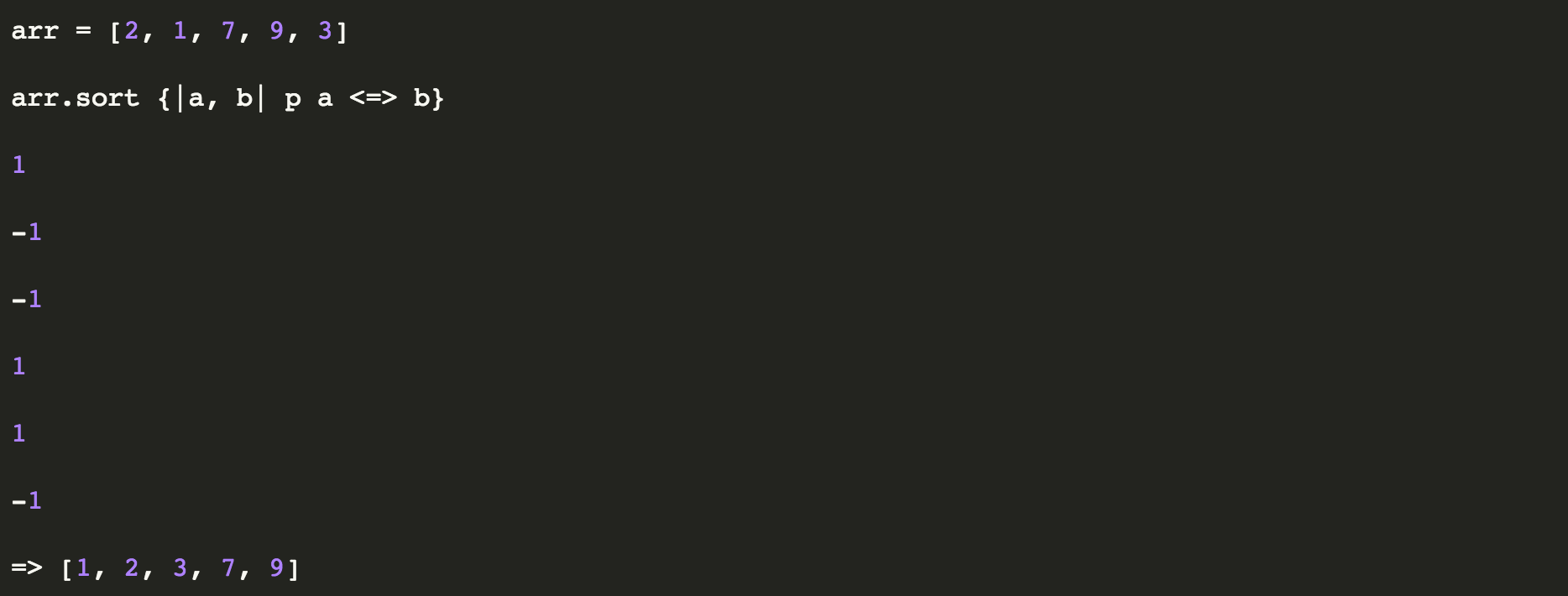`

The above example returned exactly what we expected, an array sorted in ascending order, [1, 2, 3, 7, 9]. But, how did it compare each number to get us there?

What it did was compare the left number to the right number and move the larger number to the right until it found the correct order. In our example, 2 is larger than 1 so the order was flipped in the array. The next comparison was 2 and 7, and here the elements stayed in the same position because 7 is larger than 2. Next it compared 7 to 9 and again didn’t alternate postions because 9 is larger than 7. It then compared 9 and 3, 7 and 3, and finally 2 and 3.

`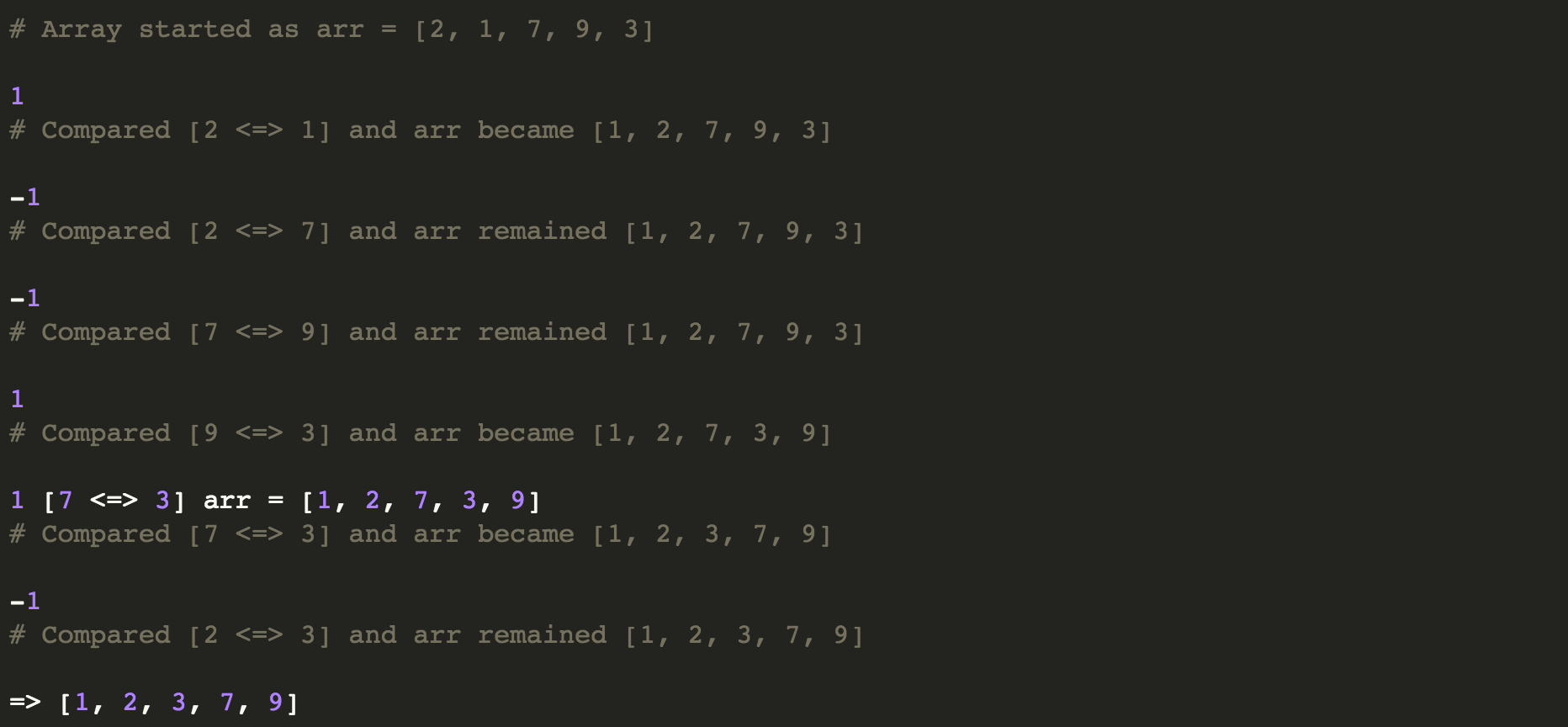`